# Prism Definition

Prism Definition
Go back to  'Geometry'

We all have drawn shapes on a piece of paper using straight lines. Now, imagine it stretching up from the sheet of paper as shown in the figure. The 3D shape so formed gives you the idea of what a prism looks like!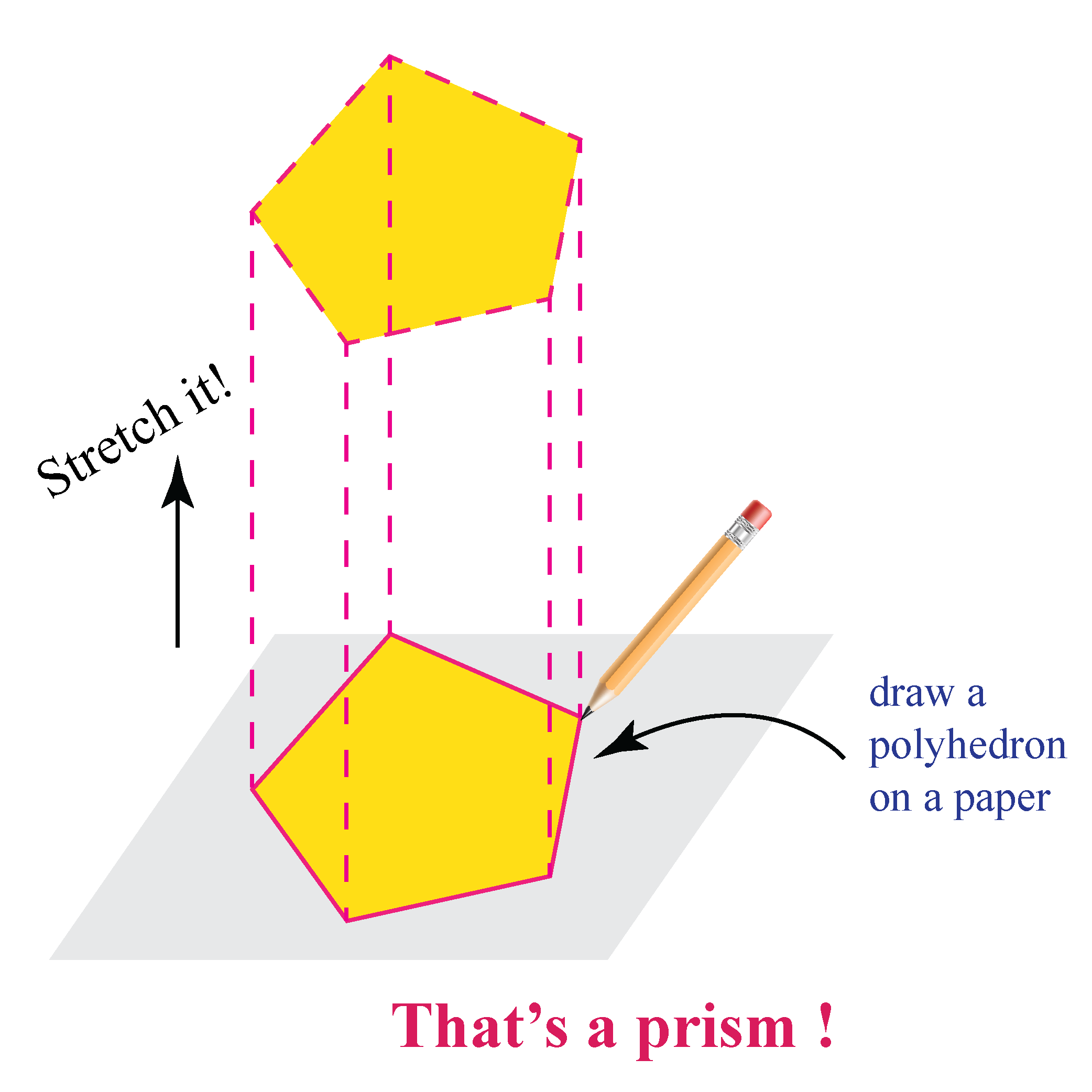In this mini-lesson, we will explore the world of prisms by finding the answers to the questions like what are prisms, definition with shapes and examples, the difference between regular, irregular, right, and oblique prisms, the surface area of a prism, and the volume of a prism with the help of interactive questions.

## What Are Prisms?

### Prism Definition In Math

A prism is a 3-dimensional solid object which has identical ends, flat faces, and the same cross-section all along its length.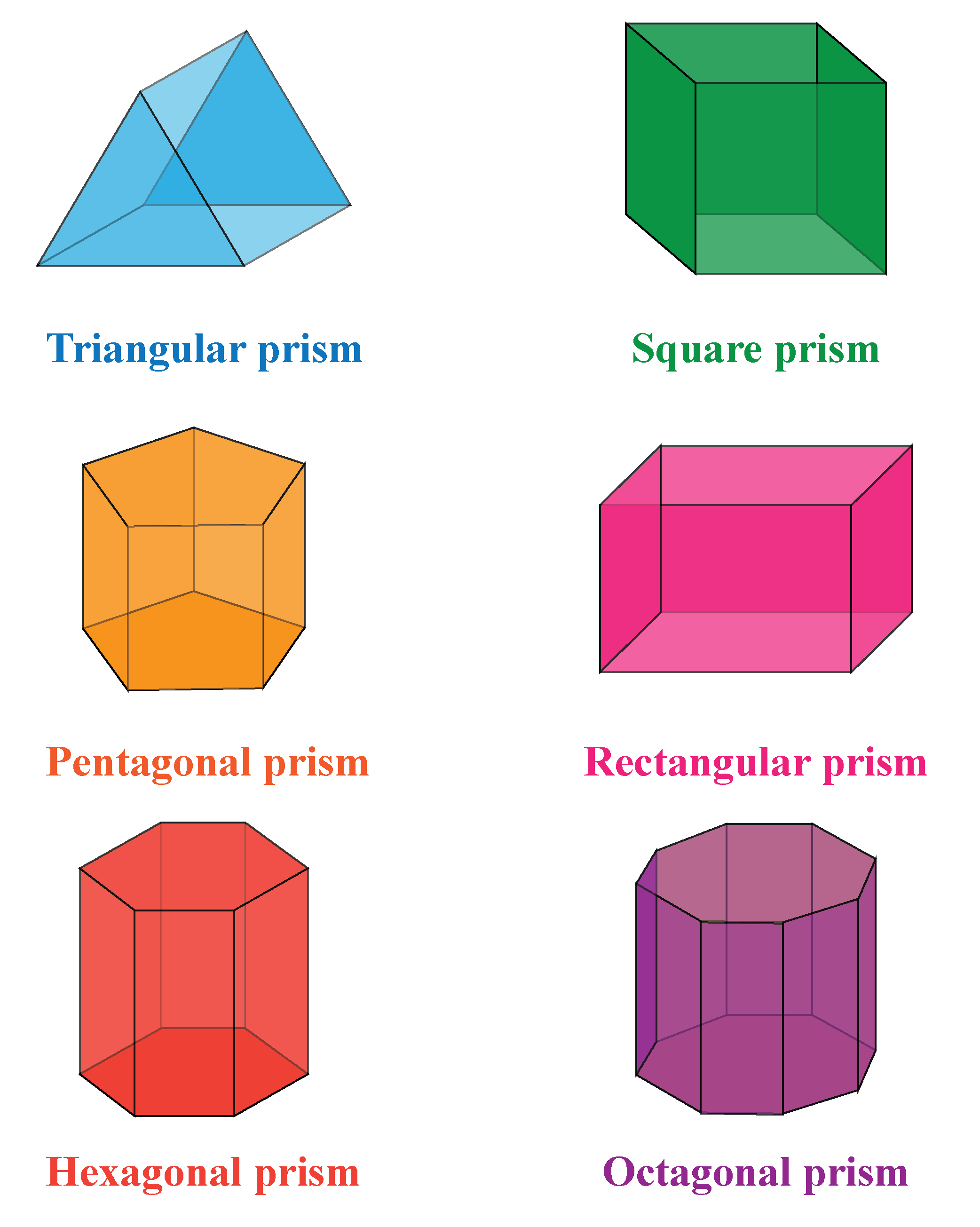A prism is a polyhedron, which means all its faces are flat. It has no curves.

For example, a cylinder is not a prism since it has curved sides.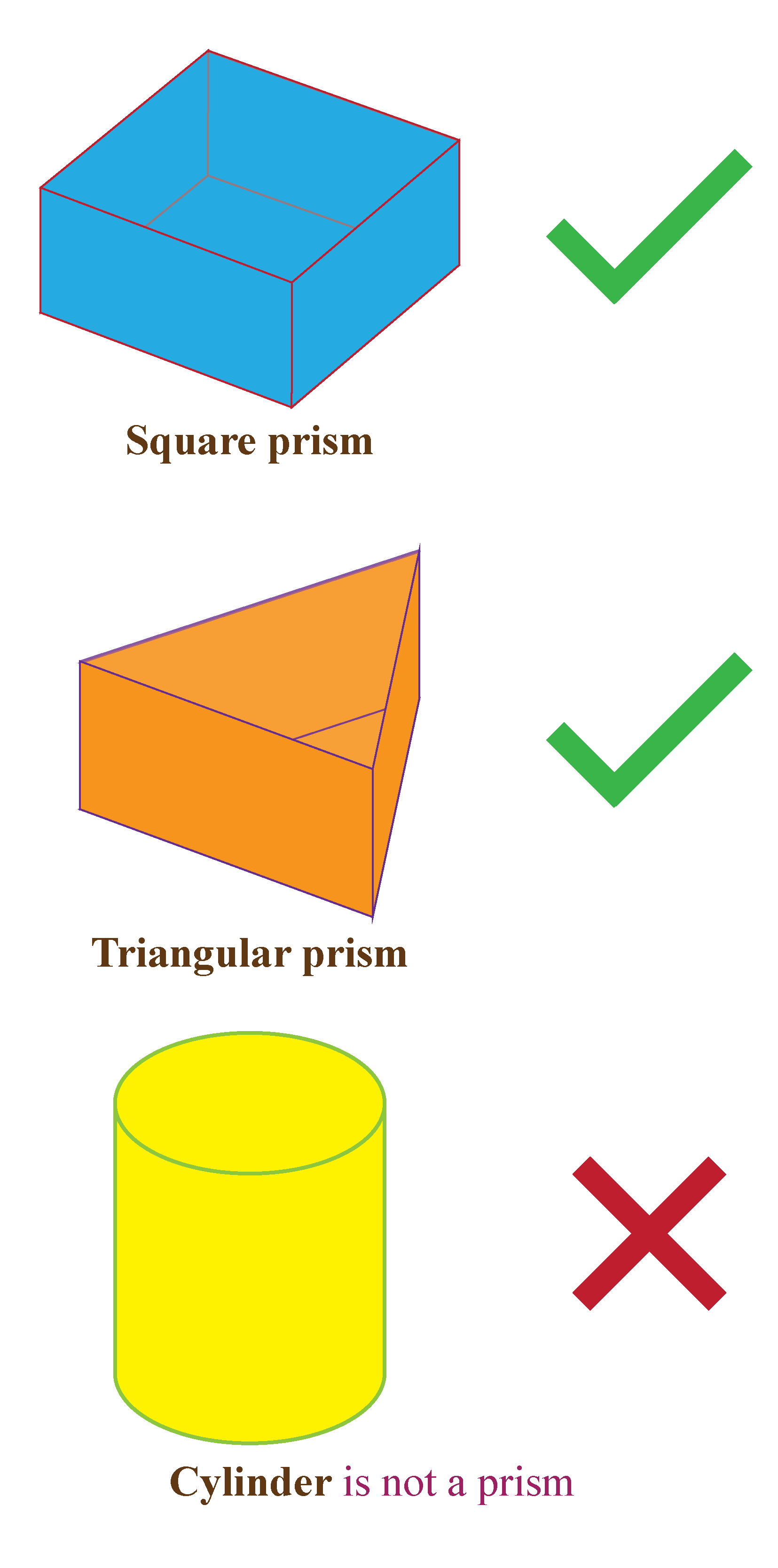## Regular Vs Irregular Prisms

The prisms are named depending upon their cross-sections. Prisms are of two types, namely:

1. Regular Prism: In a regular prism, the base of the prism is in the shape of a regular polygon.

2. Irregular Prism: In an irregular prism, the base of the prism is in the shape of an irregular polygon.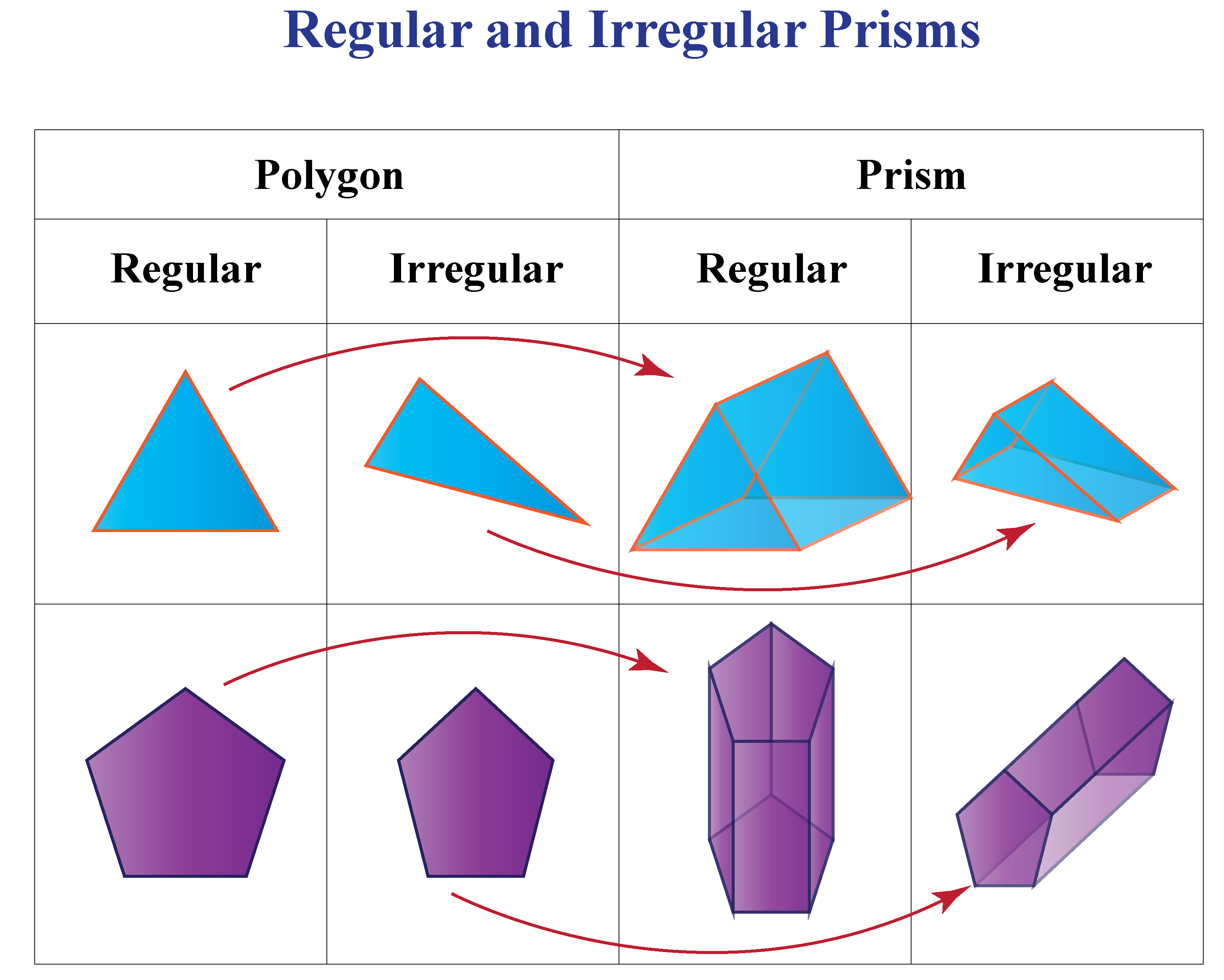## Right Vs Oblique Prisms

The two flat ends are perfectly aligned in a right prism.

An oblique prism appears to be tilted and the two flat ends are not aligned.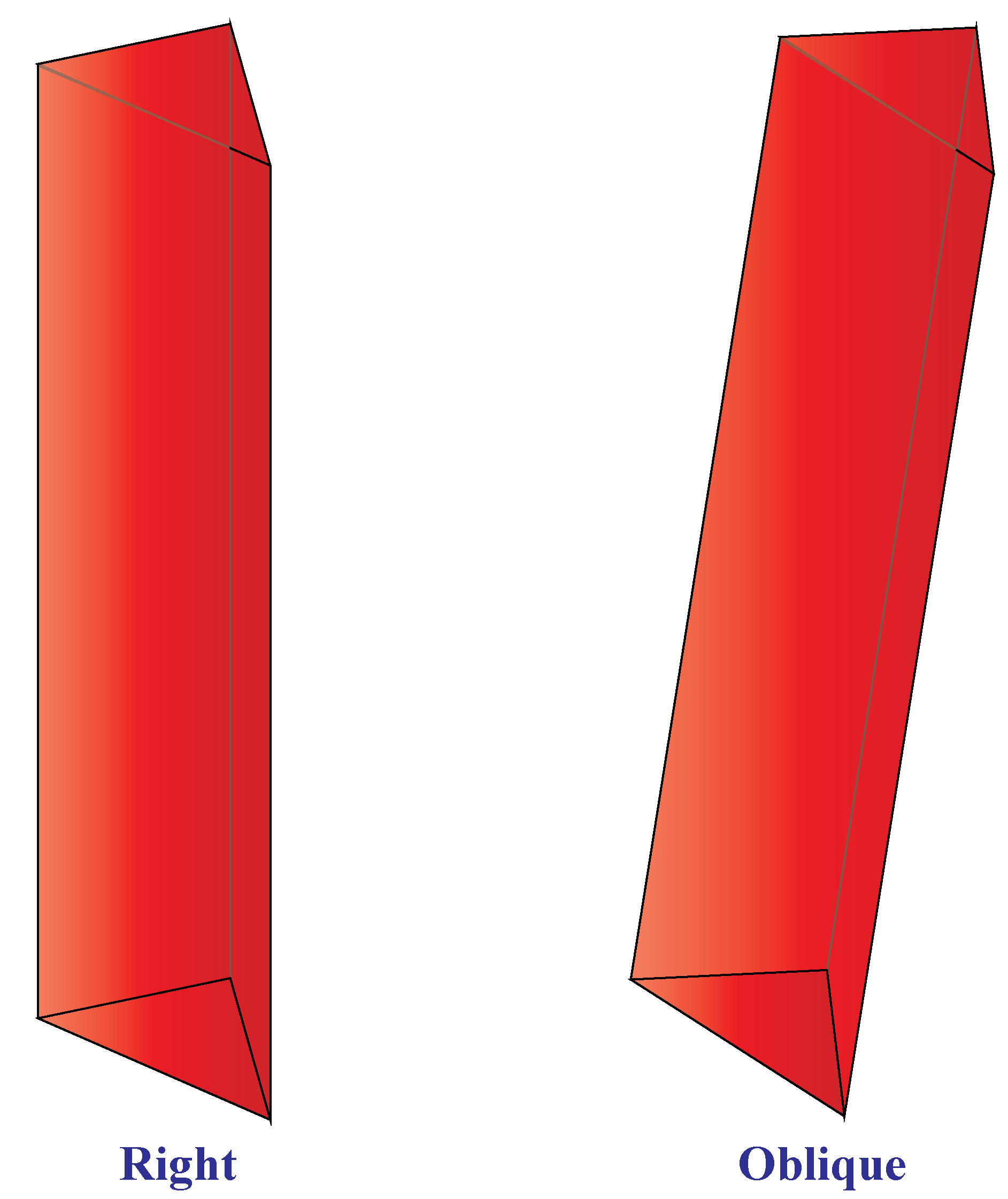## What is the Surface area of a Prism?

Prisms usually have flat faces and their cross-section is the same across its length.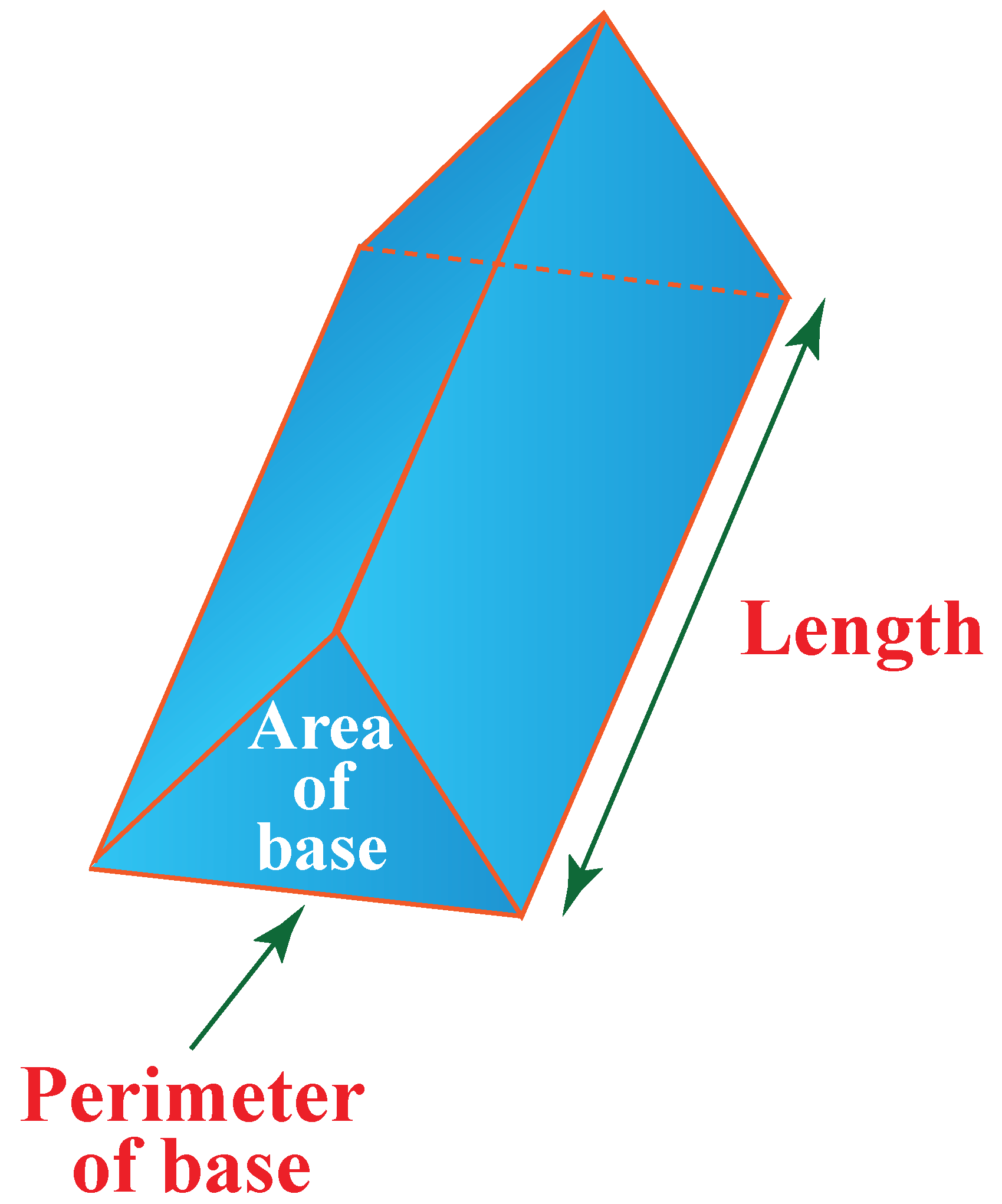They are found in regular and irregular shapes.

Based on the alignment of the apex to the center of the base, they are classified as right prisms or oblique prisms.

To find the surface area of any kind of prism we use the general formula:

 Surface area of a Prism= ($$2\times$$ Base Area)  + (Base perimeter $$\times$$ height)

## Volume of a Prism

The amount of space occupied by a 3-dimensional prism is known as its volume.

The volume is measured in cubic units.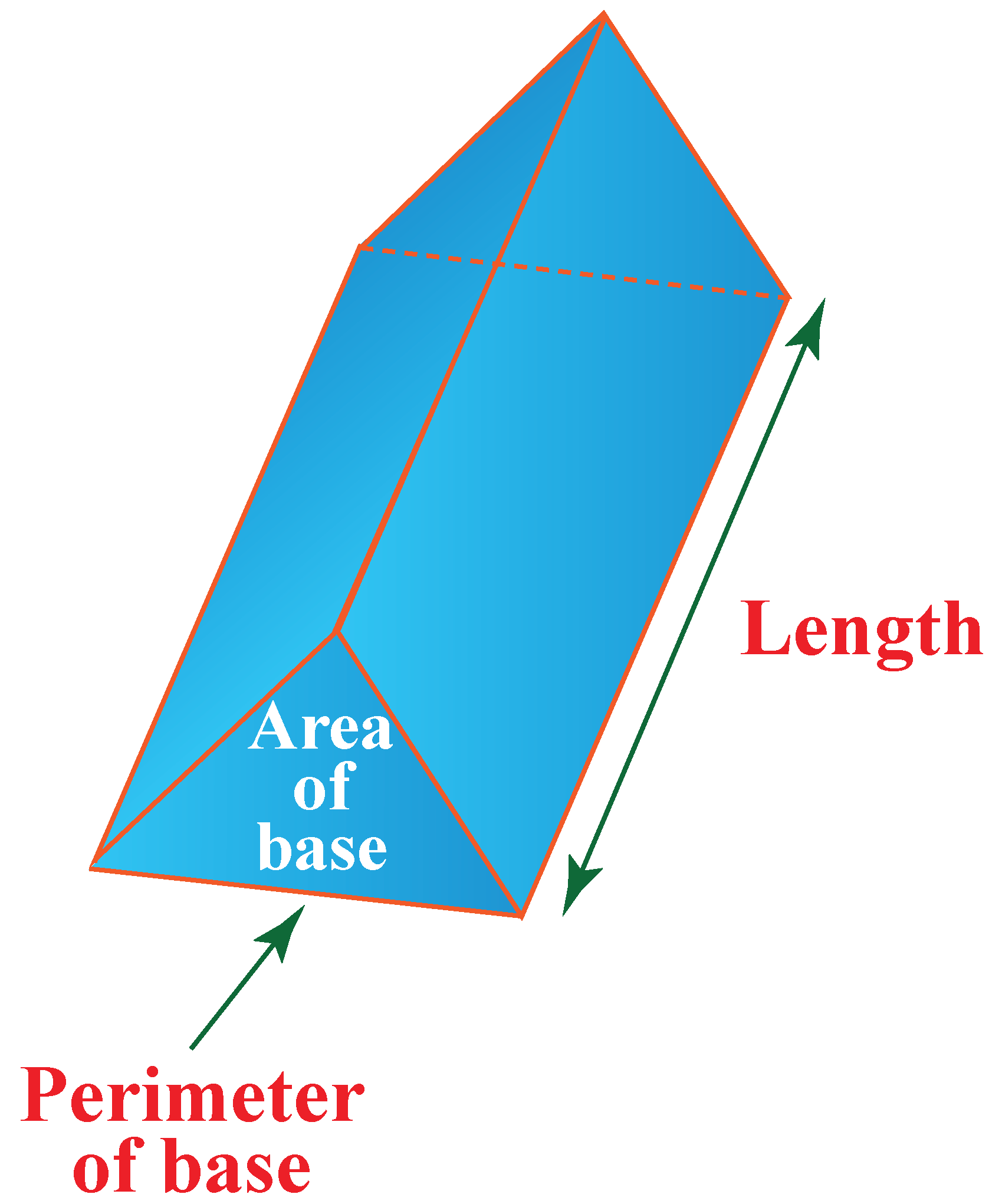The formula for the volume of a prism is:

 Volume of a Prism= ( Base Area $$\times$$ Height )Important Notes

a) A prism has the same cross-sectional area throughout its length.
b) It has identical ends (polygonal) and flat faces.
c) Surface area of a Prism is:
( $$2\times$$ Base Area)  + (Base perimeter $$\times$$ height)
d) Volume of a Prism is:
( Base Area $$\times$$ Height )
b) The volume of a prism is its cross-sectional area multiplied by its length.

## Solved Examples On Prisms

To understand the prism definition in geometry and the prism definition in math, go through the following solved examples.

 Example 1

Can you help Jamie identify the objects which resemble a rectangular prism?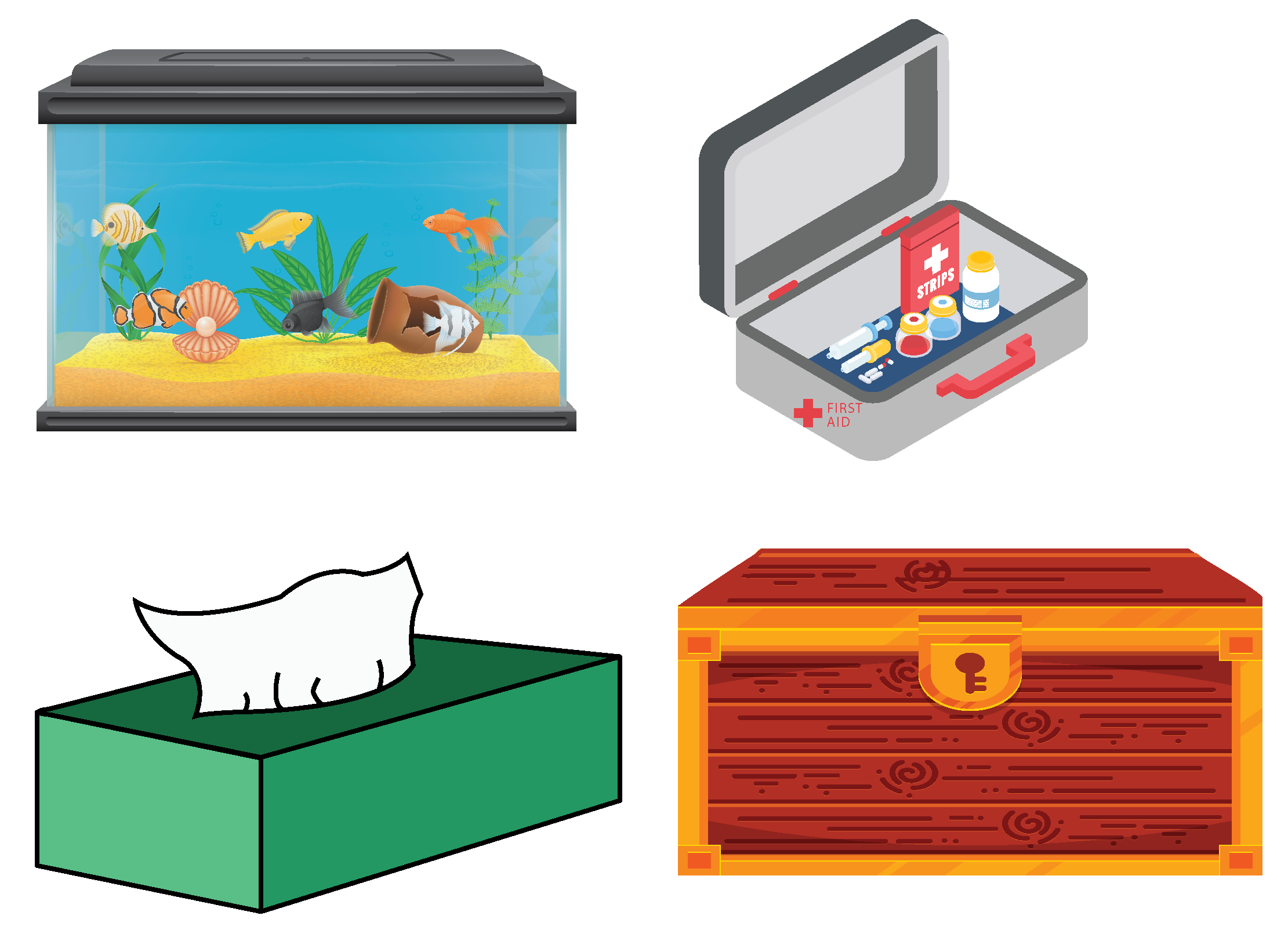Solution

To identify the rectangular prism let us discuss the properties of a rectangular prism.

• It has all six faces as rectangles.
• The opposite faces are equal.
• The cross-section along the length is the same.

Since all the above conditions are satisfied in the above images, we can say that all the four objects resemble a rectangular prism.

 $$\therefore$$ All the four objects resemble a rectangular prism.
 Example 2

Identify the pentagonal prism from the following images: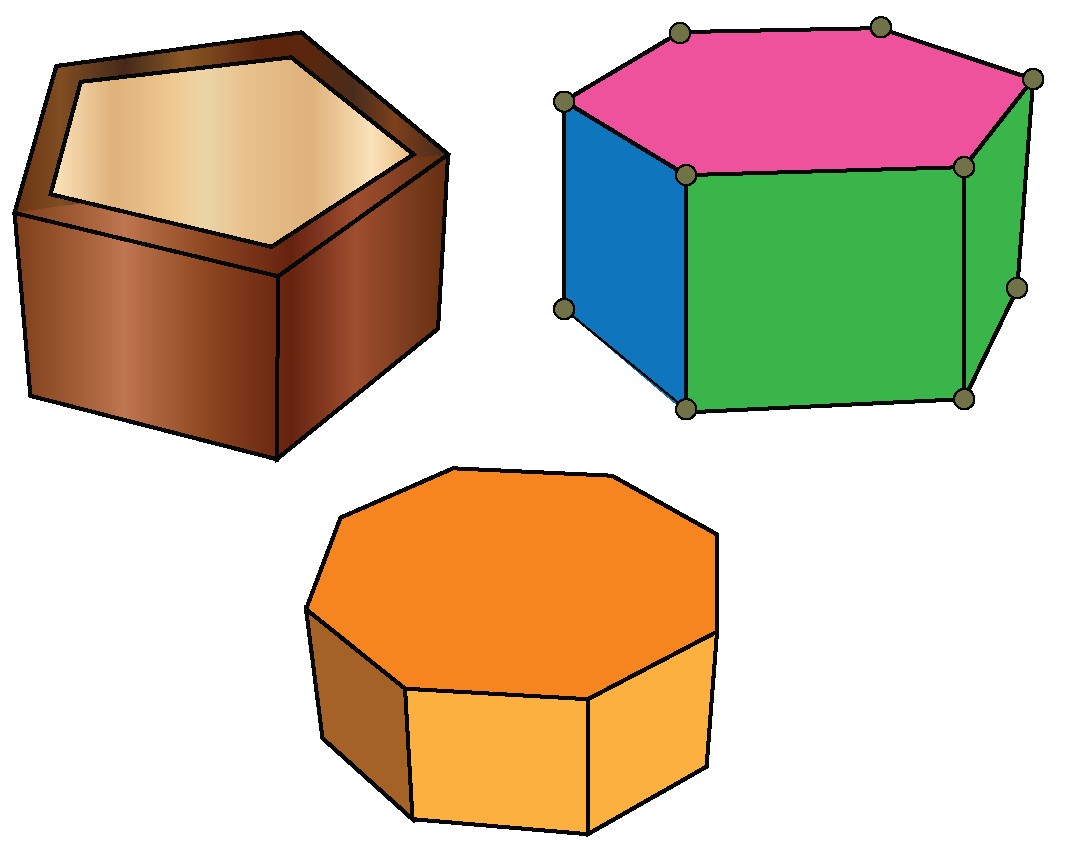Solution

The basic properties of a pentagonal prism are:

• It has 7 faces, 10 vertices, and 15 edges.
• A pentagonal prism has two pentagons as two opposite and parallel faces.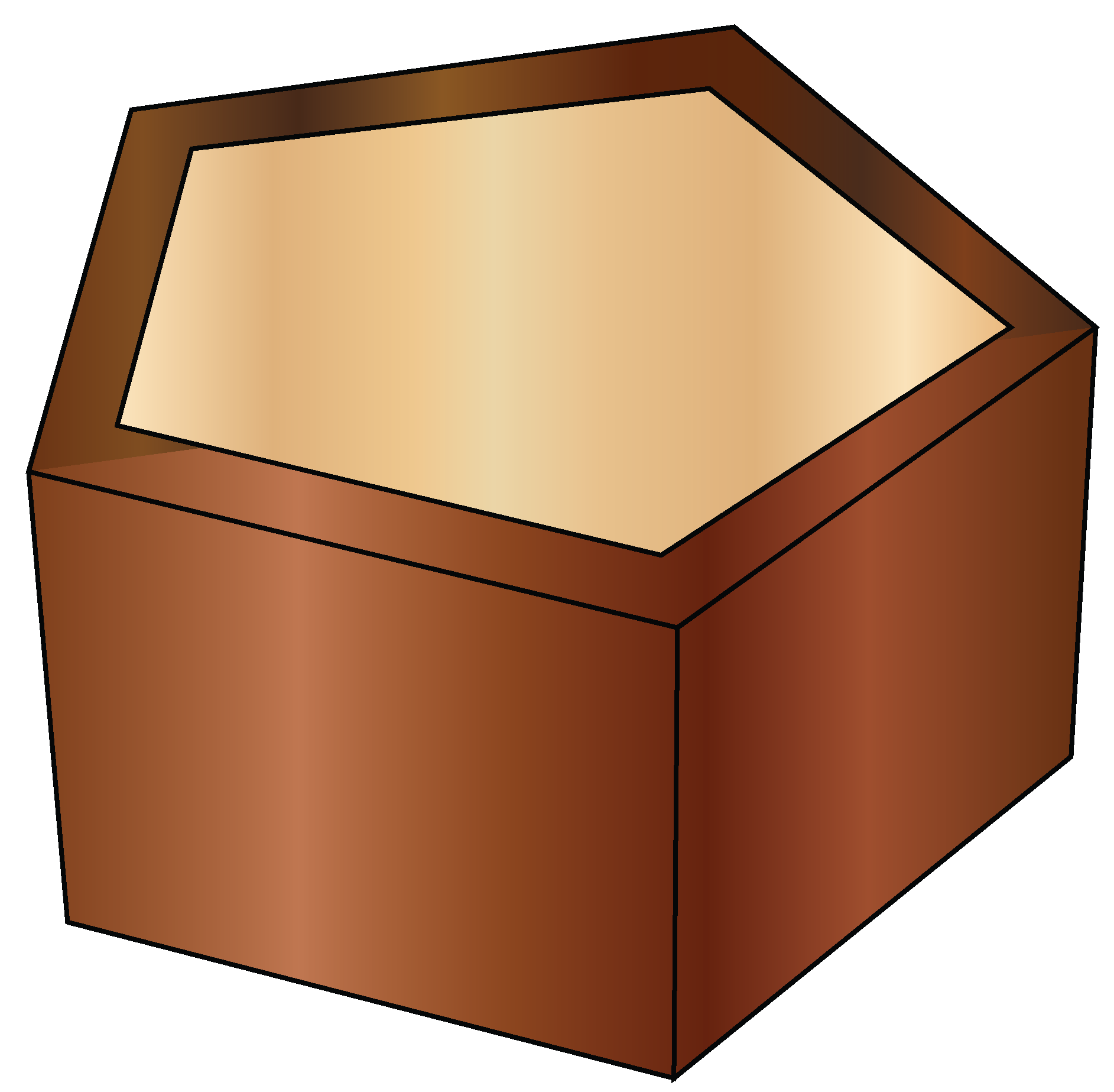The above image satisfies all the properties of a pentagonal prism.

Therefore, it is a pentagonal prism.

 $$\therefore$$ The first object is a pentagonal prism.
 Example 3

Help Anna find the volume of a triangular prism whose area is $$35\ in^2$$ and height is $$6\ in$$.

Solution

Given, base area=$$35\ in^2$$ and height=$$6\ in$$

We know that,

Volume of a Prism is

\begin{align} V &=\text{Base area}\times\text{Height}\\\ &=35\times6\\\ &=210\ in^3\end{align}

Hence, the volume of the triangular prism=$$210\ in^3$$

 $$\therefore$$  V= $$210\ in^3$$Challenging Questions
a) Find the missing values and name the prisms with the following identities:
 Faces Edges Vertices 7 x 10 x 18 12
b) Find the ratio of volumes of two triangular prisms with lengths 'a' and 'b'. Both of these prisms have the same cross-sectional area.

## Interactive Questions on Prisms

Here are a few activities for you to practice. Select/Type your answer and click the "Check Answer" button to see the result.

## Let's Summarize

The mini-lesson targeted the fascinating concept of prism definition. The math journey around the prism definition starts with what a student already knows, and goes on to creatively crafting a fresh concept in the young minds. Done in a way that not only it is relatable and easy to grasp, but also will stay with them forever. Here lies the magic with Cuemath.

At Cuemath, our team of math experts is dedicated to making learning fun for our favorite readers, the students!

Through an interactive and engaging learning-teaching-learning approach, the teachers explore all angles of a topic.

Be it worksheets, online classes, doubt sessions, or any other form of relation, it’s the logical thinking and smart learning approach that we, at Cuemath, believe in.

## 1. How do you find the cross-section of a prism?

Prisms are 3-dimensional figures. To find the cross-section of a prism, observe the shape of the prism as a 2-dimensional geometry object.

For example,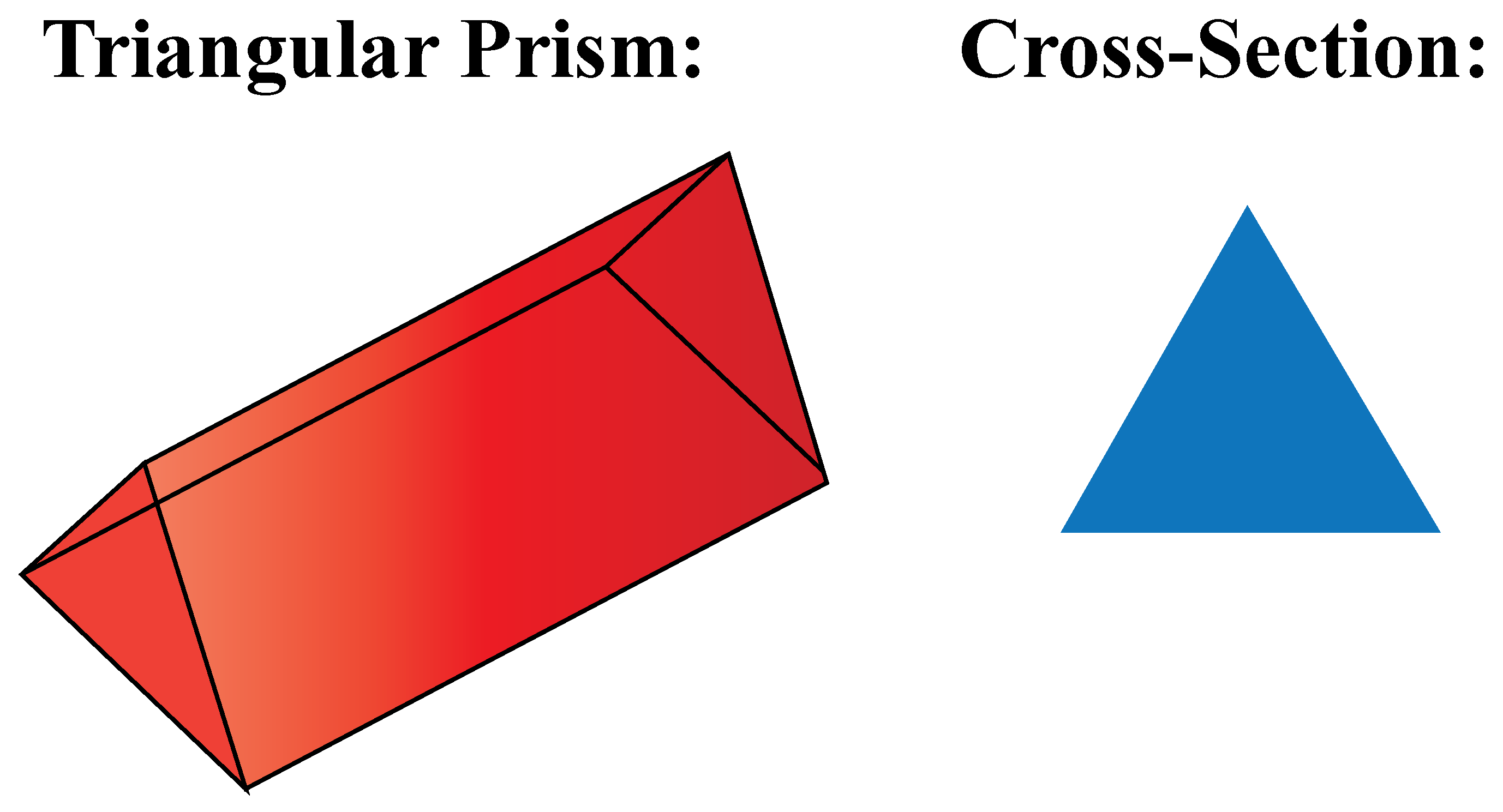## 2. What is a right Prism in math definition?

The two flat ends are perfectly aligned in the right prism.

For example,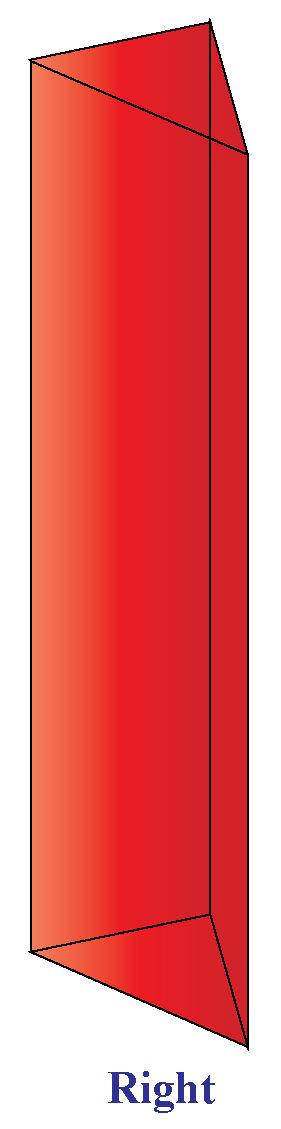## 3. What are the uses of a prism?

Prisms can be seen in many real-life, useful and beautiful articles. For example,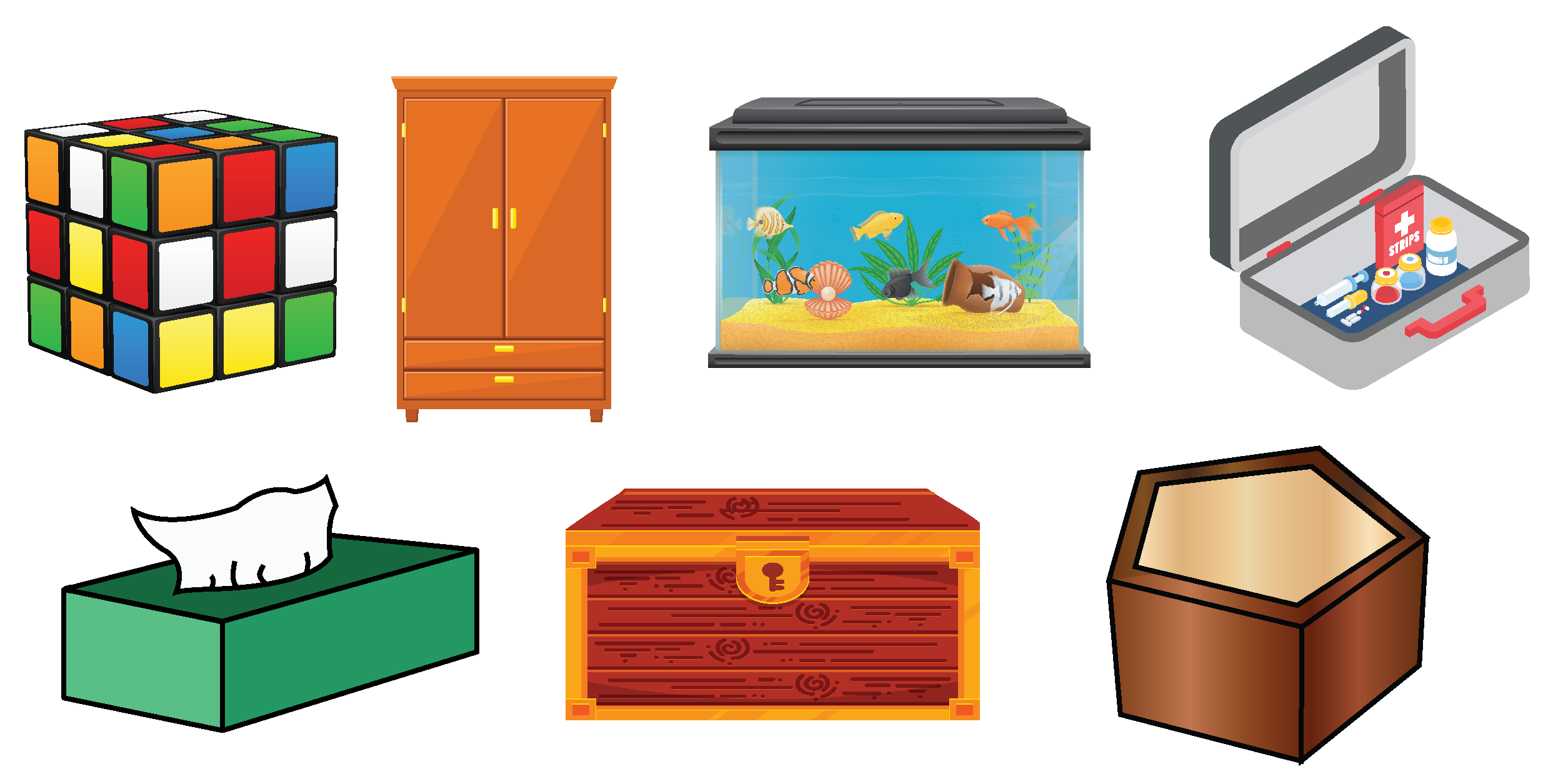More Important Topics
Numbers
Algebra
Geometry
Measurement
Money
Data
Trigonometry
Calculus